Select Page

# Combinational Logic Design

Combinational Logic Design

Contents

Describe Combinational Circuits 2

Explain Half Subtractor and Full Subtractor 4

Differentiate between Serial and Parallel Adders 9

Describe Binary Multipliers 15

Describe magnitude Comparators 15

Describe Parity Bit Generator and Checker 17

Recall Multiplexer 18

Design 2 : 1 and 4 : 1 Multiplexers 19

Design 8 : 1 and 16 : 1 Multiplexers 19

Design Multiplexer with the help of other Multiplexer 21

Recall Demultiplexers 22

Design 1 : 2 and 1 : 4 Demultiplexers 23

Design 1 : 8 Demultiplexer and 1 : 16 Demultiplexers 23

Recall Encoders 24

Design Octal to Binary Encoder and Decimal to BCD Encoder 25

Recall Decoders 26

Design Binary to Octal Decoder and BCD to Decimal Decoder 26

Design BCD to Seven Segment Decoder 27

# Describe Combinational Circuits

Combinational circuits are digital circuits that generate an output based solely on the current input values. They do not have any internal memory, and the output is determined by the logical function of the input signals at any given time. Combinational circuits are built using logic gates such as AND, OR, NOT, NAND, NOR, and XOR gates.

The simplest type of combinational circuit is a logic gate, which has one or more inputs and a single output. The output of a logic gate is determined by the logical function of the input signals. For example, an AND gate has two input signals and produces an output signal that is high only if both input signals are high.

Combinational circuits can be built by combining multiple logic gates in various ways. For example, a combinational circuit can be designed to perform arithmetic operations such as addition or subtraction, using a combination of logic gates. Other common applications of combinational circuits include data multiplexers and demultiplexers, encoders and decoders, and various types of digital signal processing.

The design of a combinational circuit involves specifying the logical function that the circuit is intended to perform, and then choosing the appropriate combination of logic gates to implement that function. The circuit can be tested using simulation software or by constructing a physical prototype.

Combinational circuits are an important building block of digital electronics and are used extensively in computer systems, telecommunications, and other digital applications. They are often used in conjunction with sequential circuits, which have internal memory and can generate outputs that depend on both the current input and the previous state of the circuit.

Half adder and full adder are combinational digital circuits that are used to perform binary addition operations. The main difference between the two circuits is that a half adder can only add two single-bit binary numbers and produce a sum and a carry bit, while a full adder can add three single-bit binary numbers and produce a sum and a carry bit.

A half adder is a combinational circuit that takes two single-bit binary numbers as input and produces two output bits: a sum bit (S) and a carry bit (C). The truth table for a half adder is shown below: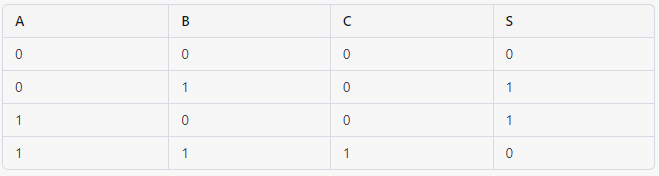The sum bit (S) is the XOR of the two input bits (A and B), while the carry bit (C) is the AND of the two input bits. A half adder can be implemented using two logic gates: an XOR gate and an AND gate.

A full adder is a combinational circuit that takes three single-bit binary numbers as input and produces two output bits: a sum bit (S) and a carry bit (C). The truth table for a full adder is shown below: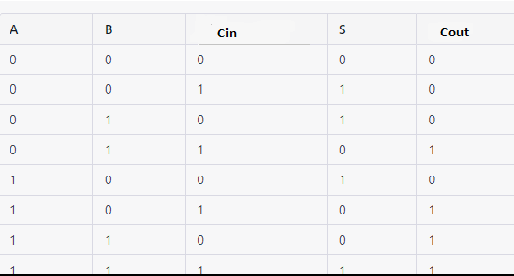The sum bit (S) is the XOR of the three input bits (A, B, and Cin), while the carry bit (Cout) is determined by the majority function of the three input bits. A full adder can be implemented using two half adders and an OR gate, or by using two XOR gates, two AND gates, and an OR gate.

In summary, a half adder is a combinational circuit that can add two single-bit binary numbers and produce a sum and a carry bit, while a full adder is a combinational circuit that can add three single-bit binary numbers and produce a sum and a carry bit. These circuits are fundamental building blocks of digital electronics and are used extensively in computer systems, telecommunications, and other digital applications.

# Explain Half Subtractor and Full Subtractor

Half Subtractor:

A half subtractor is a combinational circuit that is used to perform subtraction of two binary digits. It has two inputs, the minuend (A) and the subtrahend (B), and two outputs, the difference (D) and the borrow (Borrow out).

The difference (D) is obtained by subtracting the subtrahend (B) from the minuend (A). If A is greater than or equal to B, then the difference is equal to A-B. If A is less than B, then the difference is equal to 0 and the borrow output is set to 1.

Full Subtractor:

A full subtractor is a combinational circuit that is used to perform subtraction of two binary digits, including borrowing from the previous subtraction. It has three inputs, the minuend (A), the subtrahend (B), and the borrow input (Borrow in), and two outputs, the difference (Difference) and the borrow (Borrow out).

The difference (Difference) is obtained by subtracting the subtrahend (B) and the borrow input (Borrow in) from the minuend (A). If A is greater than or equal to B + Borrow in, then the difference is equal to A-(B + Borrow in). If A is less than B + Borrow in, then the difference is equal to 0 and the borrow output is set to 1.

A serial adder is a combinational digital circuit used to perform binary addition of two numbers bit by bit in a serial manner. In a serial adder, the bits of the two numbers are added one at a time, starting from the least significant bit (LSB) and working towards the most significant bit (MSB).

The basic architecture of a serial adder includes two inputs: one input is the number to be added (the augend), and the other input is the number to add (the addend). The circuit also includes a carry input and a carry output, which are used to propagate carries from one bit position to the next. The carry input is initially set to 0 for the first bit position, and the carry output of each bit position is connected to the carry input of the next bit position.

The addition of two bits in a serial adder is performed using a full adder. The full adder takes in three inputs: the two bits to be added (one from the augend and one from the addend) and the carry input from the previous bit position. The full adder produces two outputs: the sum bit and the carry output. The sum bit is added to the next bit position, and the carry output is propagated to the carry input of the next bit position.

The operation of a serial adder can be illustrated using the following example:

Suppose we want to add the two numbers 1101 and 1010 using a serial adder. The addition process proceeds as follows:

1 1 0 1 augend

———

0 0 0 0 initial carry in

———

1 0 0 0 sum (LSB)

———

1 0 1 0 carry out (LSB)

———

1 0 1 1 sum (bit position 2)

———

0 0 1 1 carry out (bit position 2)

———

1 1 1 1 sum (bit position 3)

———

1 1 1 0 carry out (bit position 3)

———

1 0 1 1 sum (MSB)

———

In this example, the sum of the two numbers is 10111 in binary, which is equal to 23 in decimal.

Serial adders are commonly used in microprocessors and other digital circuits where the operands are stored in registers and added bit by bit in a sequential manner. However, serial adders are slower than parallel adders, which can add multiple bits simultaneously, and are generally used only for small numbers of bits.

A ripple carry adder, also known as a parallel adder, is a type of digital circuit used to perform binary addition of two numbers in parallel, rather than bit by bit in a serial manner like a serial adder. In a ripple carry adder, the bits of the two numbers are added simultaneously, starting from the least significant bit (LSB) and working towards the most significant bit (MSB).

The basic architecture of a ripple carry adder includes two inputs: one input is the number to be added (the augend), and the other input is the number to add (the addend). The circuit also includes a carry input and a carry output, which are used to propagate carries from one bit position to the next. The carry input is initially set to 0 for the first bit position, and the carry output of each bit position is connected to the carry input of the next bit position.

The addition of two bits in a ripple carry adder is performed using a full adder. The full adder takes in three inputs: the two bits to be added (one from the augend and one from the addend) and the carry input from the previous bit position. The full adder produces two outputs: the sum bit and the carry output. The sum bit is added to the sum output of the previous bit position, and the carry output is propagated to the carry input of the next bit position.

The operation of a ripple carry adder can be illustrated using the following example:

Suppose we want to add the two numbers 1101 and 1010 using a ripple carry adder. The addition process proceeds as follows:

1 1 0 1 augend

———

1 0 0 1 sum (LSB)

———

0 1 1 1 carry out (LSB)

———

1 1 0 1 sum (bit position 2)

———

1 0 0 0 carry out (bit position 2)

———

1 0 1 1 sum (bit position 3)

———

1 1 0 0 carry out (bit position 3)

———

1 0 1 1 sum (MSB)

———

1 0 0 0 carry out (MSB)

———

In this example, the sum of the two numbers is 10111 in binary, which is equal to 23 in decimal.

Ripple carry adders are commonly used in microprocessors and other digital circuits where the operands are stored in registers and added in parallel. However, as the carry must propagate through each bit position, the speed of the ripple carry adder is limited by the number of bits being added. As a result, faster adders, such as carry lookahead adders, are often used for larger numbers of bits.

# Differentiate between Serial and Parallel Adders

Serial and parallel adders are two types of digital circuits that perform the addition of two binary numbers. The main difference between them is the way they process the addition of the binary numbers.

• A serial adder processes the binary numbers bit by bit in a serial fashion.
• It uses a full adder circuit to add each corresponding pair of bits from the two numbers and the carry bit from the previous stage.
• The outputs of the full adders are connected in a cascade fashion to form a serial adder circuit.
• The result of the addition is obtained after processing all the bits of the two numbers.
• Serial adders are simple to implement and are suitable for low-speed applications where computation time is not a critical issue.

• A parallel adder processes the binary numbers in parallel, i.e., it adds corresponding bits of the two numbers simultaneously.
• It consists of multiple full adder circuits, each of which adds three binary inputs: the corresponding bits of the two numbers and the carry input from the previous stage.
• The carry bit is propagated from one stage to the next, similar to a ripple effect, hence the name “ripple carry adder.”
• The result of the addition is obtained in a single clock cycle, making parallel adders more suitable for high-speed and high-precision applications.
• The speed of a parallel adder is limited by the slowest full adder stage, and the propagation delay can become an issue for large adder circuits.

Please note that these are general characteristics, and there may be variations and different implementations of serial and parallel adders depending on specific requirements and design choices.

A Look-Ahead Carry Adder (LACA) is a type of digital circuit used to perform binary addition of two numbers, similar to the ripple carry adder. However, unlike the ripple carry adder, which has a carry chain that propagates the carry from one bit to the next, the LACA generates the carry signals for all bit positions simultaneously. This results in a much faster addition process, especially for larger numbers of bits.

The basic architecture of an LACA includes two inputs: one input is the number to be added (the augend), and the other input is the number to add (the addend). The circuit also includes a carry input and a carry output, which are used to propagate carries from one bit position to the next. In an LACA, the carry signals are generated by a set of logic gates, rather than being propagated from one bit position to the next.

The generation of the carry signals in an LACA is based on the following two equations:

Gi = Ai AND Bi (Generate)

Pi = Ai XOR Bi (Propagate)

where Gi is the generate signal, Pi is the propagate signal, Ai is the ith bit of the augend, and Bi is the ith bit of the addend.

The generate and propagate signals are used to calculate the carry signal for each bit position using the following equation:

Ci = Gi + Pi*Ci-1

where Ci is the carry signal for the ith bit position and Ci-1 is the carry signal for the (i-1)th bit position. The carry signals are then used to calculate the sum for each bit position using the following equation:

Si = Ai XOR Bi XOR Ci-1
where Si is the sum for the ith bit position.

The generation of the carry signals in an LACA is much faster than the propagation of the carry signal in a ripple carry adder. This is because the generate and propagate signals can be calculated simultaneously for all bit positions, and the carry signals can then be calculated using these signals. As a result, the LACA is much faster than the ripple carry adder for larger numbers of bits.

Overall, the Look-Ahead Carry Adder is a more efficient way to add two binary numbers as it reduces the time required to propagate the carry signal from one bit position to the next, which can become a significant bottleneck for larger numbers of bits.

A Binary Coded Decimal (BCD) adder is a digital circuit that performs addition of two BCD numbers. BCD is a binary representation of decimal numbers, in which each decimal digit is represented by a four-bit binary code.

The basic architecture of a BCD adder is similar to that of a binary adder. It consists of a series of full adder circuits, which perform addition of each corresponding bit position of the two BCD numbers. However, in a BCD adder, the carry output from each full adder is used to adjust the result to ensure that it remains in BCD form.

The adjustment process involves checking each four-bit group of the sum to see if it represents a decimal number greater than 9 (i.e., a value greater than 1001 in binary). If the group represents a value greater than 9, the group is adjusted by adding 6 to it. This is because, in BCD representation, the binary values 1010 through 1111 are not used to represent decimal digits, and the next valid BCD value after 1001 is 1010 (decimal 10).

The adjustment process can be performed using a set of logic gates, which examine each four-bit group of the sum and determine whether an adjustment is needed. The adjusted sum is then passed on to the next stage of the BCD adder, where the process is repeated until the final result is obtained.

Overall, a BCD adder is a specialized type of digital circuit that is used to perform addition of BCD numbers. It is similar to a binary adder in structure, but it includes additional logic to ensure that the result remains in BCD form. BCD adders are used in a variety of applications, such as in calculators, cash registers, and other digital systems that require decimal arithmetic.

# Describe Binary Multipliers

A binary multiplier is a digital circuit that performs the multiplication of two binary numbers. Binary multiplication is performed by multiplying each bit of one number by the corresponding bits of the other number and then summing the results.

There are two main types of binary multipliers:

1. Array Multiplier: An array multiplier is a digital circuit that implements the multiplication of two binary numbers using an array of AND gates. The inputs to the AND gates are the binary numbers, and the outputs of the AND gates are the intermediate products. The intermediate products are then summed using a binary adder circuit to generate the final product.
2. Booth Multiplier: A Booth multiplier is a digital circuit that implements the multiplication of two binary numbers using a modified version of the array multiplier. The Booth multiplier uses a bit-serial approach, where each bit of the multiplier is processed one at a time, and uses a two’s complement representation to reduce the number of partial products. The Booth multiplier is more efficient than the array multiplier as it generates fewer partial products, reducing the number of gates and the complexity of the circuit.

In conclusion, binary multipliers are digital circuits that perform the multiplication of two binary numbers. The two main types of binary multipliers are array multipliers and Booth multipliers, with the latter being more efficient due to its bit-serial approach and reduced number of partial products.

# Describe magnitude Comparators

Magnitude comparators are digital circuits that compare two binary numbers and determine whether they are equal or which one is greater. A magnitude comparator takes two n-bit inputs, A and B, and produces three outputs: A=B, A>B, and A<B.

The operation of a magnitude comparator is based on the comparison of the individual bits of the two input numbers, starting with the most significant bit (MSB) and proceeding to the least significant bit (LSB). The comparison of each pair of bits produces a binary output, which is then used to determine the relationship between the two input numbers.

There are two types of magnitude comparators: the unsigned magnitude comparator and the signed magnitude comparator.

1. Unsigned Magnitude Comparator:

The unsigned magnitude comparator compares two unsigned binary numbers, meaning two positive integers. The comparator looks at each bit of the two numbers and compares them from left to right. The comparison is done by subtracting the bits from each other, starting from the most significant bit. If the difference between the bits is zero, the comparator moves on to the next bit. If the difference is positive, the first input is greater, and if it’s negative, the second input is greater. The output of the comparator will be A>B, A<B, or A=B depending on the result of the comparison.

The truth table for a 4-bit unsigned magnitude comparator is as follows: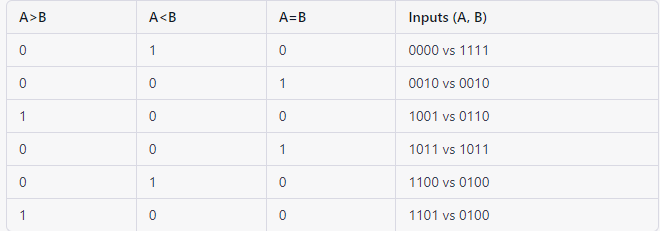1. Signed Magnitude Comparator:

The signed magnitude comparator compares two signed binary numbers, meaning two positive or negative integers. The comparator takes into account the sign bit of each input number before comparing them. If the two input numbers have the same sign, the comparator compares their magnitudes. If they have different signs, the comparator immediately determines which input number is negative, and the negative input is considered smaller than the positive input. The output of the comparator will be A>B, A<B, or A=B depending on the result of the comparison.

The truth table for a 4-bit signed magnitude comparator is as follows: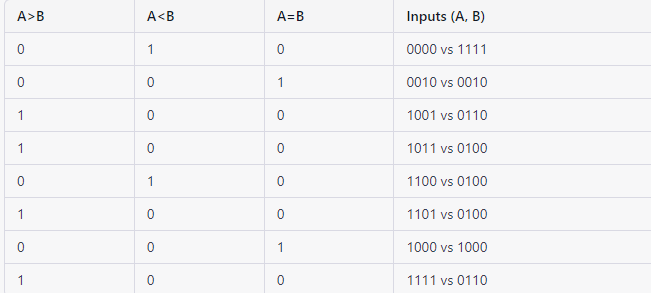# Describe Parity Bit Generator and Checker

Parity bit is a method used in error detection to ensure that data has been transmitted correctly between two devices. It works by adding an extra bit to the data that is transmitted, called the parity bit, which is used to check if the data has been received without errors.

Parity bit generator is a circuit or software algorithm that generates the parity bit for a given set of data. The generator calculates the parity bit based on the number of set bits (bits with value 1) in the data. There are two types of parity: even parity and odd parity. In even parity, the parity bit is set to 1 if the number of set bits in the data is odd, and to 0 if the number of set bits is even. In odd parity, the parity bit is set to 1 if the number of set bits in the data is even, and to 0 if the number of set bits is odd.

Parity bit checker is a circuit or software algorithm that checks if the received data is correct by comparing the parity bit with the actual number of set bits in the received data. If the parity bit does not match the number of set bits, the checker indicates an error in the received data. This allows the receiving device to request for a retransmission of the data, ensuring that the data is received without errors.

It’s worth noting that while parity bits can detect errors in data transmission, they can only detect errors in single-bit transmission errors and are not very effective in detecting multiple-bit errors or errors in the parity bit itself. For this reason, other methods such as Cyclic Redundancy Check (CRC) and checksums are used in addition to or instead of parity bits for more robust error detection.

# Recall Multiplexer

A multiplexer (also known as a MUX) is a digital circuit that selects one of several input signals and forwards the selected signal to a single output line. The selection of the input signal is controlled by a set of selection lines, also known as select lines or address lines.

A multiplexer can be thought of as a switch that selects one of its inputs to be connected to its output. The number of input signals that a multiplexer can handle is determined by the number of selection lines. For example, a 2-to-1 multiplexer has two inputs and one output, and a 4-to-1 multiplexer has four inputs and one output.

Multiplexers are commonly used in digital systems to multiplex or switch between several signals, allowing the sharing of a limited number of resources, such as communication lines or memory channels, among multiple tasks. Multiplexers are also used in data communication systems to switch between multiple communication channels, and in computer systems to select between multiple memory locations.

In digital electronics, multiplexers are commonly implemented using digital logic gates, such as AND gates, OR gates, and NOT gates. The selection of the input signal is controlled by the values of the selection lines, which determine the input signal that is connected to the output.

# Design 2 : 1 and 4 : 1 Multiplexers

A 2-to-1 multiplexer (MUX) has two inputs (A and B), one output (Z), and one select line (S). The select line determines which input is connected to the output. When the select line is set to 0, the input A is connected to the output Z, and when the select line is set to 1, the input B is connected to the output Z.

The design of a 2-to-1 multiplexer can be implemented using digital logic gates, such as AND gates, OR gates, and NOT gates. The following is one way to implement a 2-to-1 multiplexer using AND gates and OR gates:A 4-to-1 multiplexer has four inputs (A, B, C, and D), one output (Z), and two select lines (S1 and S0). The two select lines determine which input is connected to the output.

The design of a 4-to-1 multiplexer can be implemented using digital logic gates, such as AND gates, OR gates, and NOT gates. The following is one way to implement a 4-to-1 multiplexer using AND gates, OR gates, and NOT gates.It’s worth noting that the design of a 4-to-1 multiplexer can be implemented using two 2-to-1 multiplexers cascaded together, where the first 2-to-1 multiplexer selects between inputs A and B based on the value of S0, and the second 2-to-1 multiplexer selects between the outputs of the first 2-to-1 multiplexer and inputs C and D based on the value of S1.

# Design 8 : 1 and 16 : 1 Multiplexers

A 8-to-1 multiplexer (MUX) has eight inputs (A0, A1, A2, A3, A4, A5, A6, and A7), one output (Z), and three select lines (S2, S1, and S0). The three select lines determine which input is connected to the output.

The design of an 8-to-1 multiplexer can be implemented using digital logic gates, such as AND gates, OR gates, and NOT gates. One way to implement an 8-to-1 multiplexer is to cascade multiple smaller multiplexers together, such as two 4-to-1 multiplexers and four 2-to-1 multiplexers.

8-to-1 Multiplexer

In the 8-to-1 multiplexer, there are total eight inputs, i.e., A0, A1, A2, A3, A4, A5, A6, and A7, 3 selection lines, i.e., S0, S1and S2 and single output, i.e., Y. On the basis of the combination of inputs that are present at the selection lines S0, S1, and S2, one of these 8 inputs are connected to the output. The block diagram and the truth table of the 8×1 multiplexer are given below.

Block Diagram: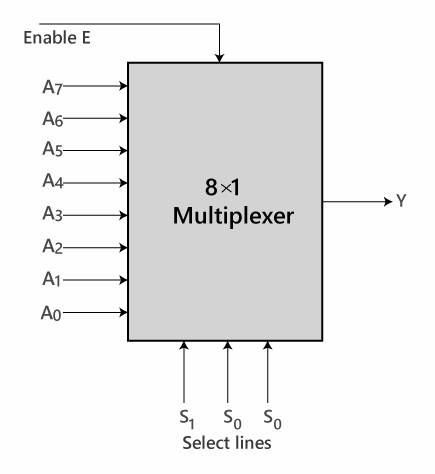16-to-1 Multiplexer

A 16-to-1 multiplexer has sixteen inputs (A0, A1, A2, …, A15), one output (Z), and four select lines (S3, S2, S1, and S0). The four select lines determine which input is connected to the output.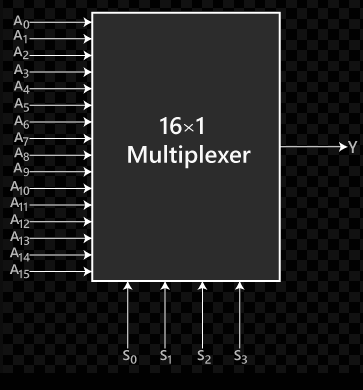# Design Multiplexer with the help of other Multiplexer

A multiplexer (MUX) can be designed by cascading smaller multiplexers together. For example, to design a 16-to-1 multiplexer, you can use four 2-to-1 multiplexers and two 4-to-1 multiplexers.

It is a combinational circuit which has many data inputs and single output depending on control or select inputs.​ For n input lines, log n (base2) selection lines, or we can say that for 2n input lines, n selection lines are required. Multiplexers are also known as “Data n selector, parallel to serial converter, many to one circuit, universal logic circuit​”. Multiplexers are mainly used to increase the amount of data that can be sent over the network within a certain amount of time and bandwidth.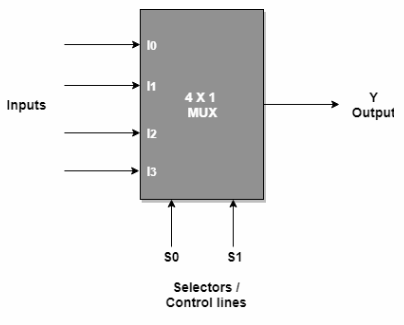# Recall Demultiplexers

A demultiplexer, also known as a data distributor or a one-to-many circuit, is a digital circuit that takes a single input data bit and routes it to one of several output lines, selected by a set of control signals. The control signals, also known as selection lines or address lines, determine which output line the data will be transmitted to. The demultiplexer is the reverse of a multiplexer, which combines multiple inputs into a single output line.

A 1:2 demultiplexer has one input line, two output lines, and one select line. A 1:4 demultiplexer has one input line, four output lines, and two select lines, and so on. The number of output lines is equal to 2n, where n is the number of selection lines.

Demultiplexers are used in digital systems to distribute a single data signal to multiple destinations, allowing parallel data processing and communication. They are used in a wide range of applications, including digital communications, computer memory, and data storage systems.

# Design 1 : 2 and 1 : 4 Demultiplexers

A demultiplexer is a device that takes a single input signal and sends it to one of many outputs. There are two main types of demultiplexers: 1:2 (binary) demultiplexers and 1:4 (quaternary) demultiplexers.

A 1:2 demultiplexer has two outputs and a single selection line. Depending on the state of the selection line, either of the two outputs will receive the input signal. For example, if the selection line is at a logic “0” state, the input signal will be sent to the first output, and if the selection line is at a logic “1” state, the input signal will be sent to the second output.

A 1:4 demultiplexer has four outputs and two selection lines. Depending on the state of the two selection lines, one of the four outputs will receive the input signal. For example, if the two selection lines are at logic “00”, the input signal will be sent to the first output, if they are at logic “01”, the input signal will be sent to the second output, and so on.

Both types of demultiplexers can be implemented using digital logic gates, such as AND gates, OR gates, and NOT gates. The specific implementation depends on the desired behaviour and the specific requirements of the system.

In summary, 1:2 and 1:4 demultiplexers are used to take a single input signal and send it to one of multiple outputs based on the state of selection lines. They can be implemented using digital logic gates, and their specific implementation depends on the desired behaviour and requirements of the system.

# Design 1 : 8 Demultiplexer and 1 : 16 Demultiplexers

A 1:8 demultiplexer is a device that has eight outputs and three selection lines. The input signal is sent to one of the eight outputs based on the state of the three selection lines. For example, if the three selection lines are at logic “000”, the input signal will be sent to the first output, if they are at logic “001”, the input signal will be sent to the second output, and so on.

A 1:16 demultiplexer is a device that has sixteen outputs and four selection lines. The input signal is sent to one of the sixteen outputs based on the state of the four selection lines. For example, if the four selection lines are at logic “0000”, the input signal will be sent to the first output, if they are at logic “0001”, the input signal will be sent to the second output, and so on.

Both 1:8 and 1:16 demultiplexers can be implemented using digital logic gates, such as AND gates, OR gates, and NOT gates. The specific implementation depends on the desired behaviour and the specific requirements of the system.

In summary, 1:8 and 1:16 demultiplexers are devices that take a single input signal and send it to one of multiple outputs based on the state of selection lines. The number of outputs and selection lines determine the specific type of demultiplexer, with 1:8 having three selection lines and 1:16 having four selection lines. They can be implemented using digital logic gates, and the specific implementation depends on the desired behaviour and requirements of the system.

# Recall Encoders

An encoder is a device that converts a set of input signals into a smaller number of coded outputs. The coded outputs represent the state of the input signals. Encoders are used in digital systems to reduce the number of input lines, simplify the logic circuits, and improve the reliability of the system.

There are two main types of encoders: binary encoders and grey code encoders. A binary encoder converts a set of binary input signals into a binary code, while a grey code encoder converts a set of binary input signals into a grey code.

A binary encoder has 2n input lines, where n is the number of bits in the output code, and produces a binary code of n bits. For example, a 2-to-1 binary encoder has two input lines and produces a single output bit, while a 4-to-2 binary encoder has four input lines and produces two output bits.

A grey code encoder also has 2n input lines and produces a grey code of n bits. The grey code is a non-weighted code in which only one bit changes at a time as the inputs change state. This helps to reduce errors in digital systems, as a change in only one bit of the code represents a change in the input signals.

In summary, encoders are devices that convert a set of input signals into a smaller number of coded outputs, representing the state of the input signals. There are two main types of encoders: binary encoders and grey code encoders, which differ in the type of code they produce. Binary encoders produce binary codes, while grey code encoders produce grey codes, which have the advantage of reducing errors in digital systems.

# Design Octal to Binary Encoder and Decimal to BCD Encoder

An octal to binary encoder is a device that converts an octal number (base-8 number system) into a binary number (base-2 number system). Octal to binary encoders typically have 8 inputs, each representing a digit in the octal number, and produce a binary number with 3 bits per octal digit.

The design of an octal to binary encoder involves mapping each possible octal digit to its corresponding binary representation. For example, the octal digit “0” could be mapped to the binary number “000”, the octal digit “1” could be mapped to the binary number “001”, and so on. This mapping can be implemented using a truth table, where each row represents a possible input combination and its corresponding output. The truth table can then be used to implement the encoder using digital logic gates, such as AND gates, OR gates, and NOT gates.

A decimal to BCD (Binary-Coded Decimal) encoder is a device that converts a decimal number (base-10 number system) into a BCD number, which is a binary representation of a decimal number. Decimal to BCD encoders typically have 10 inputs, each representing a digit in the decimal number, and produce a BCD number with 4 bits per decimal digit.

The design of a decimal to BCD encoder involves converting each decimal digit into its corresponding BCD representation. For example, the decimal digit “0” could be converted to the BCD number “0000”, the decimal digit “1” could be converted to the BCD number “0001”, and so on. This conversion can be implemented using a truth table, where each row represents a possible input combination and its corresponding output. The truth table can then be used to implement the encoder using digital logic gates, such as AND gates, OR gates, and NOT gates.

In summary, an octal to binary encoder is a device that converts an octal number into a binary number, while a decimal to BCD encoder is a device that converts a decimal number into a BCD number. Both types of encoders can be designed using a truth table and digital logic gates. The specific implementation depends on the desired behaviour and the specific requirements of the system.

# Recall Decoders

A decoder is a digital circuit that takes an n-bit input and generates a 2n output lines, with only one output line being active at a time based on the input value. There are several types of decoders, but one common type is the binary decoder, which converts a binary input into a single active output line.

In a binary decoder, each input line corresponds to a binary bit position, and the active output line represents the decimal equivalent of the binary input value. For example, a 3-to-8 decoder has three input lines and eight output lines, with each output line representing one of the decimal numbers from 0 to 7.

There are two types of binary decoders: active-high and active-low. In an active-high decoder, the output line is active (i.e., at logic level 1) when the input value matches the corresponding binary value. In an active-low decoder, the output line is active (i.e., at logic level 0) when the input value matches the corresponding binary value.

Another type of decoder is the BCD (binary coded decimal) decoder, which is used to convert a BCD input value (i.e., a decimal digit in binary form) into a corresponding output value. BCD decoders typically have four input lines and ten output lines, with each output line representing one of the decimal digits from 0 to 9.

Decoders are commonly used in digital systems to select one of several output lines based on an input value, such as in memory address decoding, data selectors, and demultiplexers. They are also used in combination with other digital circuits, such as multiplexers and encoders, to perform more complex operations.

# Design Binary to Octal Decoder and BCD to Decimal Decoder

A binary to octal decoder is a device that converts a binary number (base-2 number system) into an octal number (base-8 number system). Binary to octal decoders typically have 3 input bits per octal digit, and produce 8 outputs, each representing a digit in the octal number.

The design of a binary to octal decoder involves mapping each possible binary number to its corresponding octal digit. For example, the binary number “000” could be mapped to the octal digit “0”, the binary number “001” could be mapped to the octal digit “1”, and so on. This mapping can be implemented using a truth table, where each row represents a possible input combination and its corresponding output. The truth table can then be used to implement the decoder using digital logic gates, such as AND gates, OR gates, and NOT gates.

A BCD (Binary-Coded Decimal) to decimal decoder is a device that converts a BCD number into a decimal number (base-10 number system). BCD to decimal decoders typically have 4 input bits per decimal digit, and produce 10 outputs, each representing a digit in the decimal number.

The design of a BCD to decimal decoder involves mapping each possible BCD number to its corresponding decimal digit. For example, the BCD number “0000” could be mapped to the decimal digit “0”, the BCD number “0001” could be mapped to the decimal digit “1”, and so on. This mapping can be implemented using a truth table, where each row represents a possible input combination and its corresponding output. The truth table can then be used to implement the decoder using digital logic gates, such as AND gates, OR gates, and NOT gates.

# Design BCD to Seven Segment Decoder

A BCD (Binary Coded Decimal) to seven-segment decoder is a circuit that takes a 4-bit BCD input and converts it into the appropriate combination of output signals to drive a seven-segment display.

Here’s one possible design for a BCD to seven-segment decoder:

1. Implement the truth table: A truth table can be used to specify the desired behaviour of the decoder. It lists all possible inputs and the corresponding outputs.
2. Implement using logic gates: The truth table can be used to implement the decoder using logic gates such as AND, OR, and NOT.

Similarly, the logic expressions for the other outputs can be derived from the truth table.

1. Implement using a decoder IC: A decoder IC such as the 74LS47 or the 74LS48 can be used to simplify the design and reduce the number of logic gates required. These ICs have the truth table implemented inside and take the BCD input as input and provide the segment outputs as output.